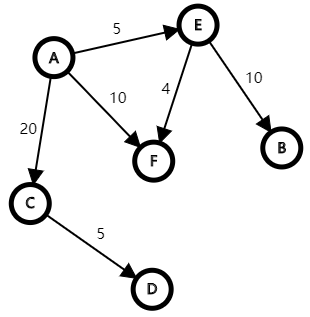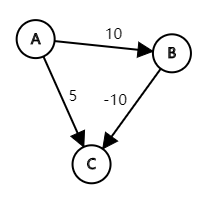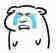# Dijkstra

dijkstra是一个运用了贪心思想和广度优先搜索的单源最短路算法，它的时间复杂度比SPFA的时间复杂度稍低，很适合在没有负边权且不是随机数据（卡SPFA）的情况下使用。

dijkstra运用了贪心的策略，将每个点与起点的距离存到一个dis数组里，若这个点不与起点直接相连则将其的距离看做是无穷大（在这里用$0x7fffffff$（int的最大值）代替）。将已经处理好的（确定是最短路的）顶点标记在一个数组里。

## 上图开讲ABCDEF
dis$0$$\infty$$20$$\infty$$5$$10 我们遍历一遍dis数组，找出离A最近的点E，并把它标记为已经处理好的点（绿色）。 ABCDEF dis0$$\infty$$20$$\infty$$\color{#52C41A}{5}$$10$

ABCDEF
dis$0$$\color{Red}{15}$$20$$\infty$$\color{#52C41A}{5}$$10 然后遍历到F点，可得A-B-F的距离（9）小于A-F的距离（10），于是更新dis数组中A-F的距离 ABCDEF dis0$$\color{Red}{15}$$20$$\infty$$\color{#52C41A}{5}$$\color{Red}{9}$

ABCDEF
dis$0$$\color{Red}{15}$$20$$\infty$$\color{#52C41A}{5}$$\color{#52C41A}{9} 遍历与F点相连的点并更新距离 没有与F点相连的点，遍历dis数组找下一个 标记B点为确定的点 ABCDEF dis0$$\color{#52C41A}{15}$$20$$\infty$$\color{#52C41A}{5}$$\color{#52C41A}{9}$

ABCDEF
dis$0$$\color{#52C41A}{15}$$\color{#52C41A}{20}$$\infty$$\color{#52C41A}{5}$$\color{#52C41A}{9} 遍历与C点相连的点并更新距离 找到D点，20+5<\infty，更新A点到D点的距离为25 ABCDEF dis0$$\color{#52C41A}{15}$$\color{#52C41A}{20}$$\color{Red}{25}$$\color{#52C41A}{5}$$\color{#52C41A}{9}$

ABCDEF
dis$0$$\color{#52C41A}{15}$$\color{#52C41A}{20}$$\color{#52C41A}{25}$$\color{#52C41A}{5}$$\color{#52C41A}{9} 所有的点都更新完了，我们就得到了A点到所有点的最短路径长度 起点终点长度 AA0 AB15 AC20 AD25 AE5 AF9 这就是利用Dijkstra寻找单源最短路径的方法。 ## 为啥不能用在带负边权的图上呢 那为什么Dijkstra不能用在带有负边权的图上呢？ 让我们用一个图来解释一下以A点为起点，最初的dis数组是这样的 ABC dis0$$10$$5 如果按照Dijkstra的步骤来，第一步我们会把C点确定 ABC dis0$$10$$\color{#52C41A}{5}$

Dijkstra真是个难学的算法## 模板

void dijkstra()
{
memset(dis,127/3,sizeof(dis));//初始化
v=1;
dis=0;
for(int i=1;i<=n;++i)
{
int k=0;
for(int j=1;j<=n;++j)//找出距离最近的点
if(!v[j]&&(k==0||dis[j]<dis[k]))
k=j;
v[k]=1;//加入集合
for(int j=1;j<=n;++j)//松弛
if(!v[j]&&dis[k]+a[k][j]<dis[j])
dis[j]=dis[k]+a[k][j];
}
}

using namespace std;
const int maxn=250;
struct edge{
int to,dis,next;
//定义边的结构体
};
edge e[maxn*2]; //边的链表
bool vis[maxn];
int n,a,b;

inline void add_edge(int u,int v,int d){
cnt++;//把边加进去
e[cnt].dis=d;
e[cnt].to=v;
}

struct node{
int dis;//点的结构体，dis为A点到这个点的距离
int pos;
bool operator <(const node &x)const
{
return x.dis<dis;//重载运算符，要不然c++没法比
}
};

priority_queue<node> q;

inline void dij(){
dis[a]=0;
q.push((node){0,a});
while(!q.empty()){
node tmp=q.top();
q.pop();
int x=tmp.pos,d=tmp.dis;
if(vis[x]) continue;
vis[x]=1;
}